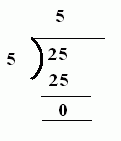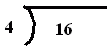Name: ___________________Date:___________________

 Email us to get an instant 20% discount on highly effective K-12 Math & English kwizNET Programs!

### MEAP Preparation - Grade 4 Mathematics1.80 Division using '')''

 Division can be written horizontally as 25/5 or 25 ÷ 5 Division problem may be written vertically as 5)25(Method: Divide the dividend by the divisor. The result is the quotient. Answer: 5 Directions: Divide the following. Also write at least 10 examples of your own.
 Q 1: Solve the following division problem.9786 Q 2: Solve the following division problem.5869 Q 3: Solve the following division problem.3486 Q 4: Solve the following division problem.8796 Q 5: Solve the following division problem.448243 Q 6: Solve the following division problem.6978 Q 7: Solve the following division problem.390109 Q 8: Solve the following division problem.9678 Question 9: This question is available to subscribers only! Question 10: This question is available to subscribers only!